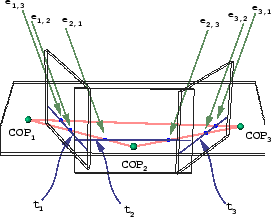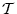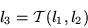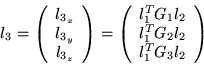Next: Application to Motion Up: Linear Approaches Previous: Epipolar Geometry

## The Trifocal Tensor

The next natural step from the stereo formalism and the fundamental matrix is a multi-camera situation (i.e. 3 or more projections). The trifocal tensor approach is such an extension and maintains a similar projective geometry spirit. This model has been proposed and developed by Sashua , Hartley  and Faugeras  among others. Figure 5 represents the imaging scenario.Here, the trifocal plane is formed by the three optic centers COP1, COP2 and COP3. Intersecting this plane with the three image planes produces three lines called the trifocal lines t1, t2 and t3. There are now two epipoles (the ei,j). One could use standard epipolar geometry and consider three fundamental matrices (one for each pair of COPs) F12, F23 and F31. However, the fundamental matrices are subject to some standard limitations which might be avoidable here. For instance, if a point P is in the trifocal plane, the fundamental matrices cannot determine if its 3 images belong to a single 3D point. In fact, there is additional information in the three plane case. Given a point in one image, it is possible to construct a line in another using the fundamental matrix. However, given a point in the first image and a point in the second image, one can directly compute the coordinates of the third point using a structure called the trifocal tensor which is the analog of the fundamental matrix for 3 view situations. Typically, one uses this tensor (denoted) to map a line in image 1 (l1) and a line in image 2 (l2) to a line in image 3 (l3). This mapping is again a linear expression as in Equation 5.(5)

To map points, one merely considers intersections of mapped lines. The tensorcan be considered as a 3 x 3 x 3 cube operator (i.e. defined by 27 scalars in total). It can also be represented as the concatenation of three 3 x 3 matrices: G1, G2 and G3 which allow us to expand the above into the more straightforward Equation 6.(6)

If a set of corresponded points are known in each of the 3 images, the tensor can be estimated in a similar way as the fundamental matrix. For instance, one can perform a least-squares linear computation to recover the 27 parameters  . However, the trifocal tensor's 27 scalar parameters are not all independent unknowns. Not every 3 x 3 x 3 cube is a tensor. It too has constraints (like the fundamental matrix) and really has only 18 degrees of freedom. The above linear methods for recovering the tensor do not impose the constraints and can therefore produce invalid tensors.

By making an appeal to Grassmann-Cayley algebra, Faugeras gracefully derives the algebraic constraints on trifocal tensors which can be viewed as higher order (4th degree) polynomials on the parameters . The 9 constraints are folded into a nonlinear optimization scheme which recovers the 18 remaining degrees of freedom of the tensor from image correspondences.Next: Application to Motion Up: Linear Approaches Previous: Epipolar Geometry

1999-05-17